PromQL（Prometheus Query Language）为Prometheus tsdb的查询语言。是结合grafana进行数据展示和告警规则的配置的关键部分。

counter（计数器） gauge （仪表类型） histogram（直方图类型） summary （摘要类型） 便于读者实践，本文大部分样本数据target：

Prometheus node_exporter

# 表达式数据类型

PromQL查询语句即表达式，实现的四种数据类型：

## Instant vector

Instance vector（瞬时向量）表示一个时间序列的集合，但是每个时序只有最近的一个点，而不是线。

## Range vector

Range vector（范围向量）表示一段时间范围里的时序，每个时序可包含多个点

## Scalar

Scalar（标量）通常为数值，可以将只有一个时序的Instance vector转换成Scalar。

• =：与字符串匹配
• !=：与字符串不匹配
• =~：与正则匹配
• !~：与正则不匹配

# 运算符

Prometheus中的运算符与各类编程语言中的基本一致。

## 数学运算符

Prometheus 中存在以下数学运算符：

• +（加法）
• -（减法）
• *（乘法）
• /（除法）
• %（取模）
• ^（幂） 两个标量之间的计算 瞬时向量与标量计算，由于计算后值意义与原指标名有差异，Prometheus很贴心的帮我们移除了指标名称。 两个瞬时向量间的计算，如下计算node的内存使用率

group_left以左侧为准

## 比较运算符

Prometheus 中存在以下比较运算符：

• ==（相等）
• !=（不相等）
• >（大于）
• <（小于）
• >=（大于或等于）
• <=（小于或等于）

## 逻辑运算符

Prometheus 中存在以下逻辑运算符：

• and（与）
• or（或）
• unless（非）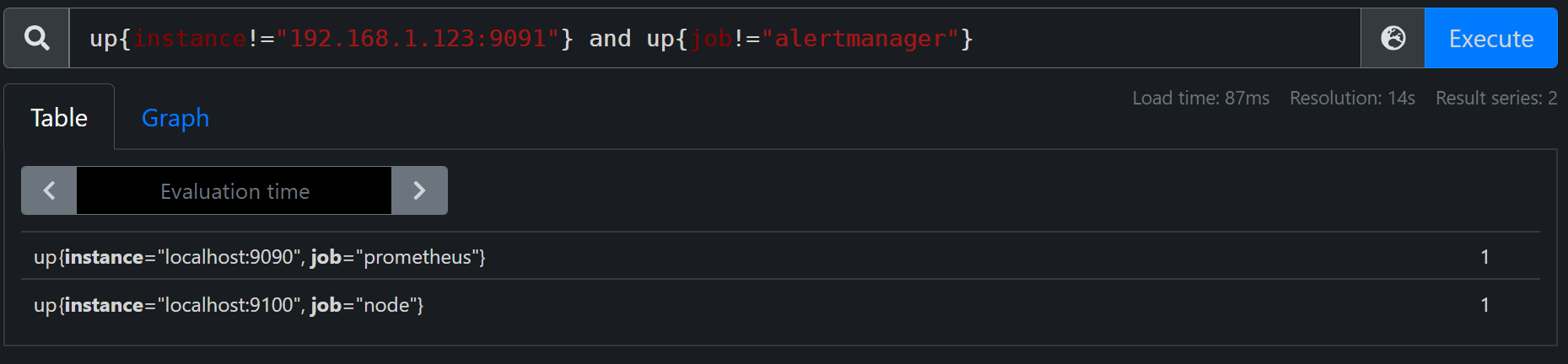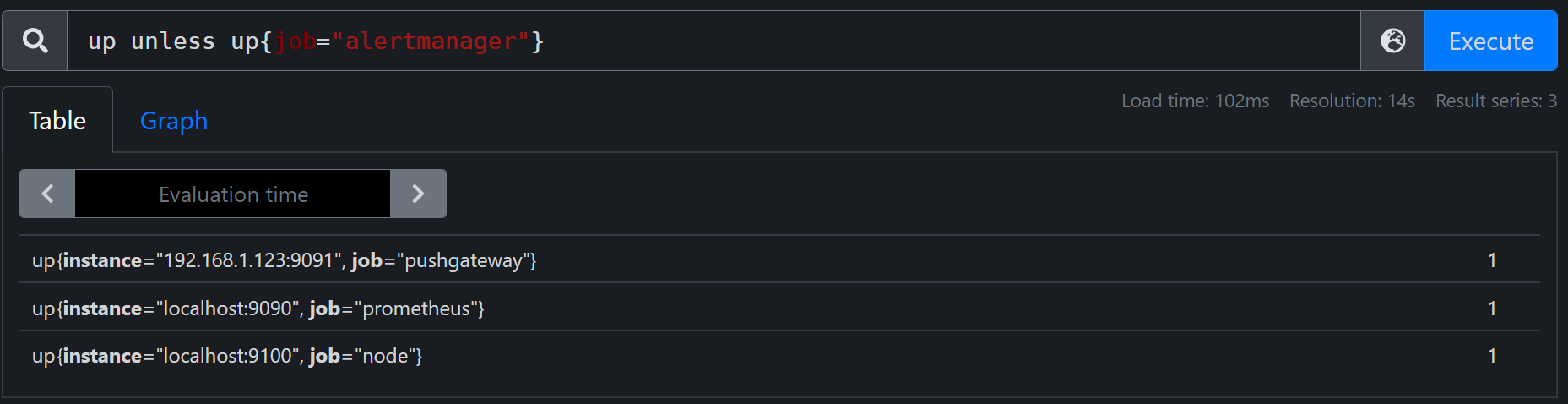Prometheus 中二元运算符的优先级，从高到低。

1. ^
2. *, /, %,atan2
3. +,-
4. ==, !=, <=, <, >=,>
5. and,unless
6. or

## 聚合运算符

Prometheus 支持以下内置聚合运算符，可用于聚合单个瞬时向量，生成新的向量：

• sum（总和）
• min（最小）
• max（最大
• avg（平均值）
• group（分组）
• stddev（标准偏差）
• stdvar（标准方差）
• count（计算向量中的元素个数）
• count_values（计算具有相同值的元素个数）
• bottomk（样本值的最小 k 个元素）
• topk（按样本值计算的最大 k 个元素）
• quantile（分位数计算 φ-quantile (0 ≤ φ ≤ 1)
• 聚合运算符可通过 without、by 根据标签扩展

sum、min、max、avg：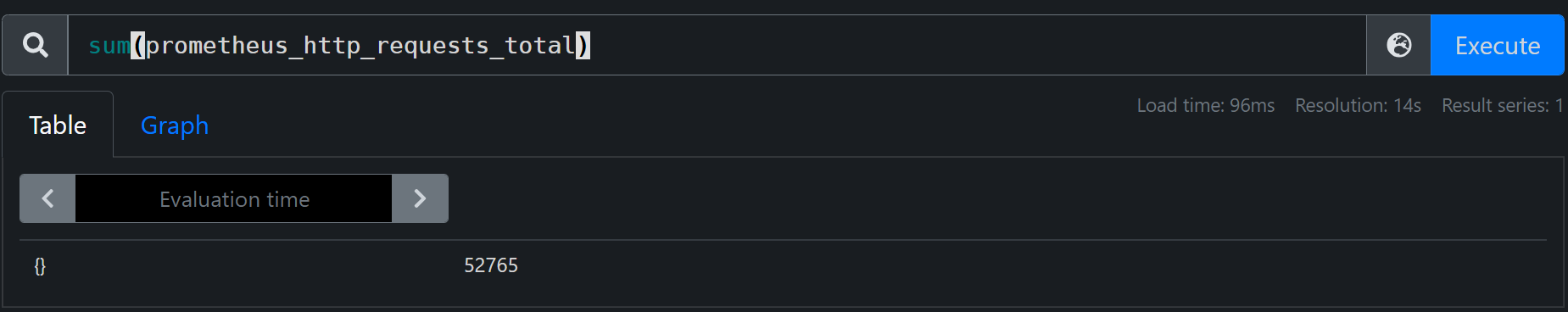通过状态码分别统计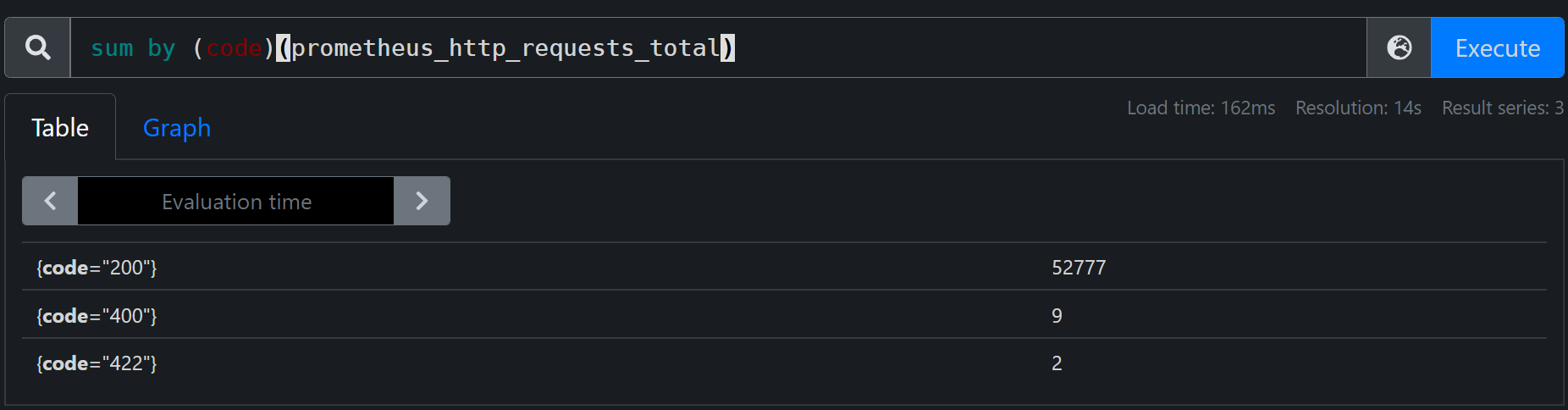group:

stddev、stdvar：

rate计算某段时间的速率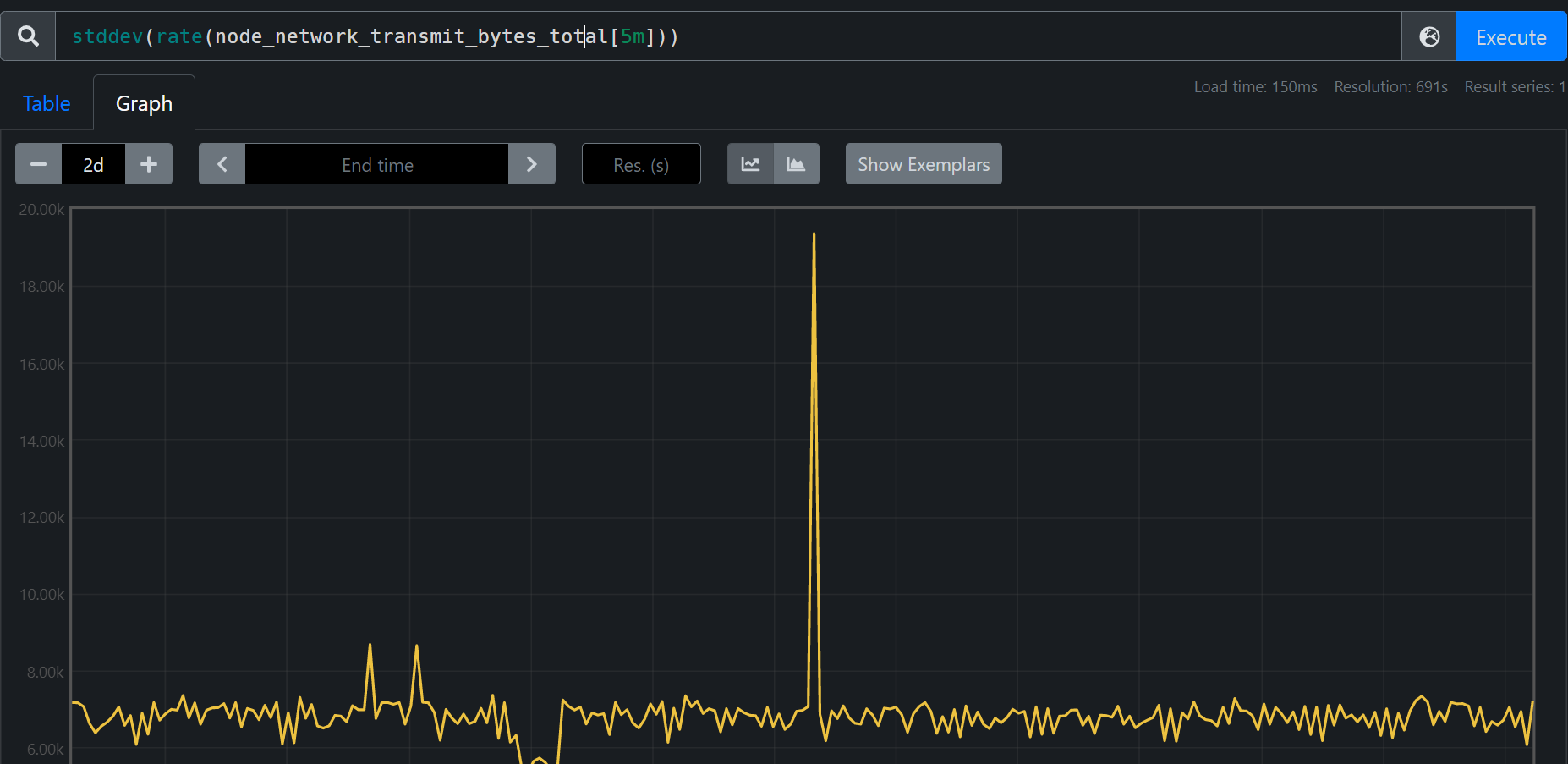count、count_values: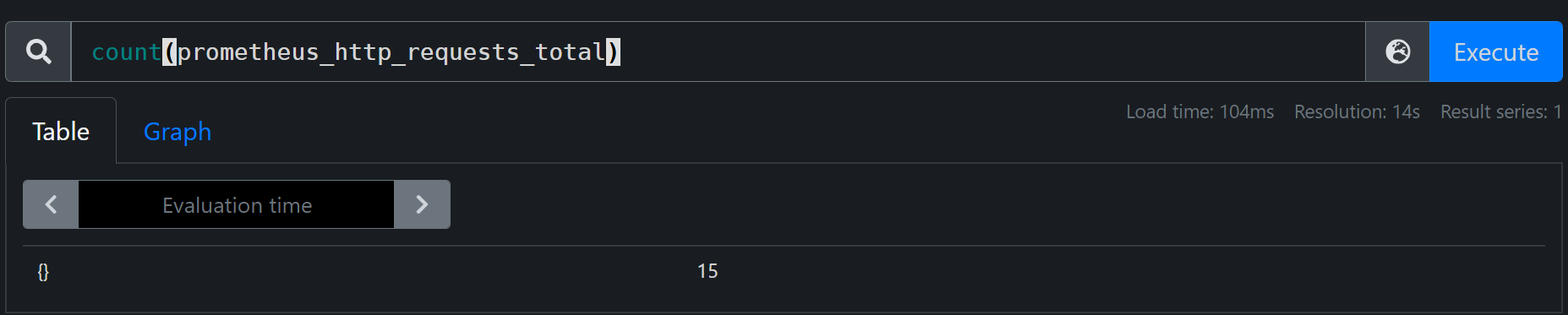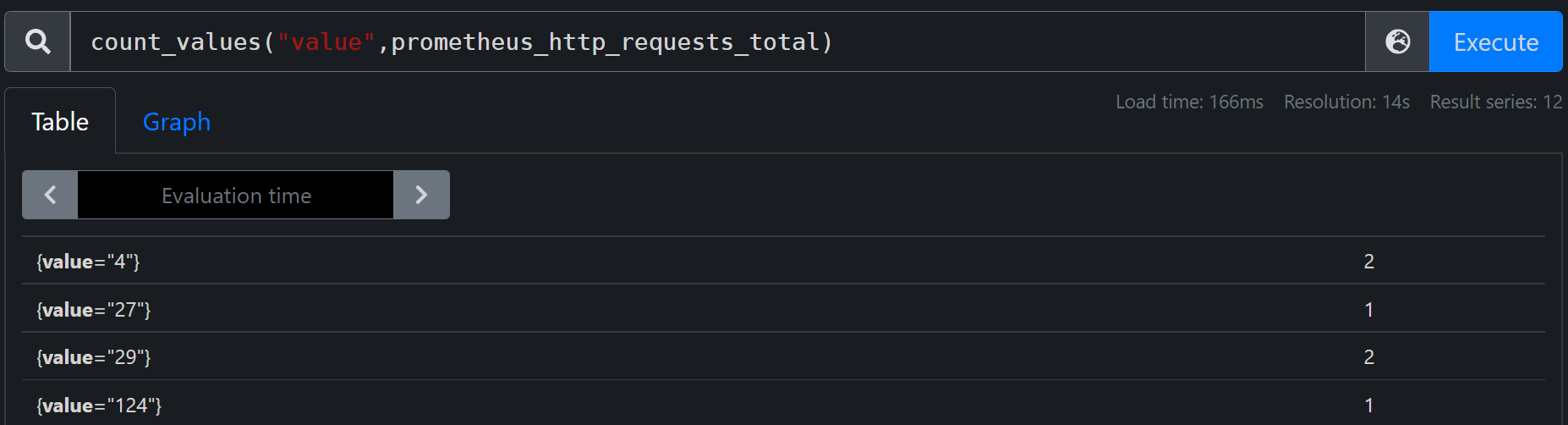bottomk、topk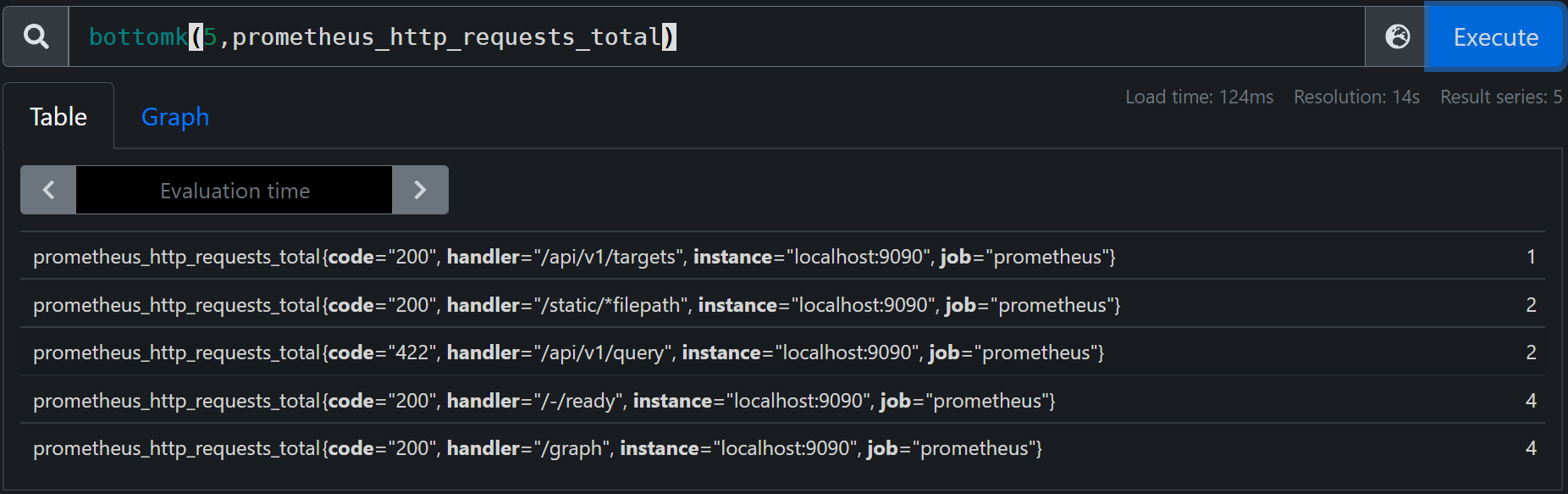quantile:求数据的分位数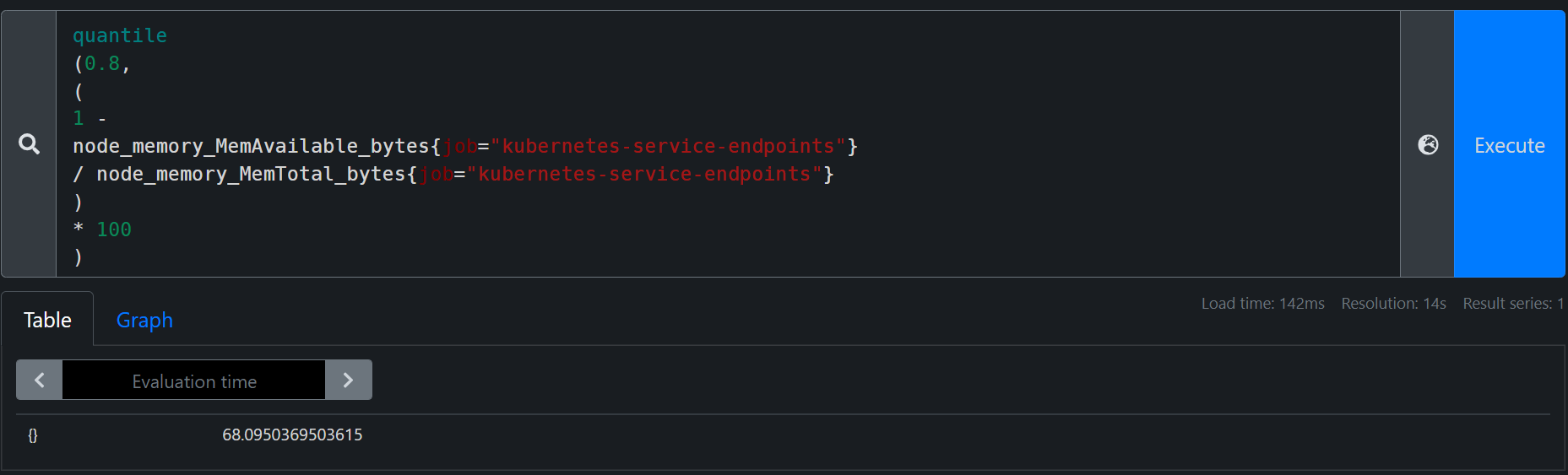直接可以看出80%的节点内存使用率在68%以下

# 函数

## 值取整

ceil()

ceil(v instant-vector)样本数据向上取整。

floor()

floor(v instant-vector)与ceil()相反，floor()样本值向下取整。

round()

round(v instant-vector, to_nearest=1 scalar) 对样本值四舍五入取整。to_nearest 参数是可选的,默认为 1,表示样本返回的是最接近 1 的整数倍的值，参数可以为分数。

## 值截取

clamp()

clamp(v instant-vector, min scalar, max scalar) 截取所有元素的样本值在 [min,max]集合内的样本,如果min>max返回NaN

clamp_max()

clamp_max(v instant-vector, max scalar) 同clamp()，不过只限定样本最大值

clamp_min()

clamp_min(v instant-vector, min scalar) 同clamp()，不过只限定样本最小值

## 值变化统计

changes()

changes(v range-vector)返回某段时间内样本值改变的次数

## 复位统计

resets()

resets(v range-vector) 返回样本范围时间内的复位次数。与counter使用，两个连续样本之间值如有减少则被视为计数器复位。

## 日期与时间管理

day_of_month()

day_of_month(v=vector(time()) instant-vector)如果样本值是utc时间，则返回这个时间所属月份中的日期（1-31）

v=vector(time()) 为默认参数

day_of_week()

day_of_week(v=vector(time()) instant-vector) 同上，如果样本值是utc时间，则返回这个时间所属星期几（0-6）

days_in_month()

days_in_month(v=vector(time()) instant-vector) 如果样本值是utc时间，则返回这个时间所属月份的天数（28-31）

hour()

hour(v=vector(time()) instant-vector)如果样本值是utc时间，则返回这个时间所属一天中的第几个小时（1-13）

minute()

minute(v=vector(time()) instant-vector) 如果样本值是utc时间，则返回这个时间所属小时中的第几分钟（1-59）

month()

month(v=vector(time()) instant-vector)如果样本值是utc时间，则返回这个时间所属的月份（1-12）

year()

year(v=vector(time()) instant-vector)如果样本值是utc时间，则返回这个时间所属的年份

time()

timestamp()

timestamp(v instant-vector)返回每个样本值的时间戳，自 1970 年 1 月 1 日 UTC 以来的秒数。

## 直方图分位数

histogram_quantile()

histogram_quantile(φ float, b instant-vector) 从 bucket 类型的向量 b 中计算 φ (0 ≤ φ ≤ 1) 分位数的样本的最大值，与聚合运算符quantile相似。

## 差异与增长率

delta()

delta(v range-vector)计算范围向量中每个时间序列元素的第一个值和最后一个值之间的差。与指标类型gauge一起使用

idelta()

idelta(v range-vector)计算范围向量中最后两个样本之间的差异。与指标类型gauge一起使用

increase()

increase(v range-vector) 计算时间范围内的增量，与counter一起使用。它是速率rate(v)乘以时间范围内秒数的语法糖，主要用于人类可读性。

rate()

rate(v range-vector)计算范围向量中时间序列的平均每秒增长率。

irate()

irate(v range-vector) 通过时间范围的最后两个点来计算每秒瞬时增长率。

## label管理

label_join()

label_join(v instant-vector, dst_label string, separator string, src_label_1 string, src_label_2 string, …)为每个时间序列添加一个label，值为指定旧label的value连接

label_replace()

label_replace(v instant-vector, dst_label string, replacement string, src_label string, regex string)从源label中获取value元素用于添加新的label

\$1 获取正则匹配，匹配值添加到hello标签中

## 预测

predict_linear()

predict_linear(v range-vector, t scalar) 通过简单线性回归预测t秒后的样本值，与gauge一起使用。

## 转换

absent()

absent(v instant-vector)如果向量有元素，则返回一个空向量；如果向量没有元素，则返回值为 1。

absent_over_time()

absent_over_time(v range-vector)如果范围向量有元素，则返回一个空向量；如果范围向量没有元素，则返回值为 1。

scalar()

scalar(v instant-vector)以标量形式返回该单元素的样本值,如果输入向量不是正好一个元素，scalar将返回NaN.

vector()

vector(s scalar)将标量作为没有标签的向量返回。

sgn()

sgn(v instant-vector)返回一个向量，其中所有样本值都转换为1或-1或0

## 排序

sort()

sort(v instant-vector)返回按样本值升序排序的向量元素。

sort_desc()

_over_time() 下面的函数列表允许传入一个范围向量，返回一个带有聚合的瞬时向量：

• avg_over_time(range-vector): 区间向量内每个度量指标的平均值。
• min_over_time(range-vector): 区间向量内每个度量指标的最小值。
• max_over_time(range-vector): 区间向量内每个度量指标的最大值。
• sum_over_time(range-vector): 区间向量内每个度量指标的求和值。
• count_over_time(range-vector): 区间向量内每个度量指标的样本数据个数。
• quantile_over_time(scalar, range-vector): 区间向量内每个度量指标的样本数据值分位数，φ-quantile (0 ≤ φ ≤ 1)
• stddev_over_time(range-vector): 区间向量内每个度量指标的总体标准偏差。
• stdvar_over_time(range-vector): 区间向量内每个度量指标的总体标准方差

## 数学函数

abs()

abs(v instant-vector) 返回样本的绝对值。

sqrt()

sqrt(v instant-vector)计算样本值的平方根。

deriv()

deriv(v range-vector) 使用简单线性回归计算时间序列在范围向量中的每秒导数。与指标类型gauge一起使用

exp()

exp(v instant-vector)计算样本值的指数函数。

Exp(+Inf) = +Inf Exp(NaN) = NaN ln()、log2()、log10()

ln/log2/log10(v instant-vector) 计算样本值对数

ln(+Inf) = +Inf ln(0) = -Inf ln(x < 0) = NaN ln(NaN) = NaN holt_winters()

holt_winters(v range-vector, sf scalar, tf scalar)基于访问向量v，生成时间序列数据平滑数据值。平滑因子sf越低, 对旧数据越重要。趋势因子tf越高，更关心趋势数据。0<sf,tf<=1。 与gauge一起使用

• acos(v instant-vector)
• acosh(v instant-vector)
• asin(v instant-vector)
• asinh(v instant-vector)
• atan(v instant-vector)
• atanh(v instant-vector)
• cos(v instant-vector)
• cosh(v instant-vector)
• sin(v instant-vector)
• sinh(v instant-vector)
• tan(v instant-vector)
• tanh(v instant-vector)

• deg(v instant-vector)
• pi()